## A minimally configured Windows laptop

November 22, 2020 Windows No comments , , , , , ,

I’ve now installed enough that my new Windows machine is minimally functional (LaTex, Linux, and Mathematica), with enough installed that I can compile any of my latex based books, or standalone content for blog posts.  My list of installed extras includes:

• Brother HL-2170W (printer driver)
• Windows Terminal
• GPL Ghostscript (for MaTeX, latex labels in Mathematica figures.)
• Wolfram Mathematica
• Firefox
• Chrome
• Visual Studio
• Python
• Julia
• Discord
• OBS Studio
• MikTeX
• SumatraPDF
• GVim
• Git
• PowerShell (7)
• Ubuntu
• Dropbox

Some notes:

• On Windows, for my LaTeX work, I used to use MikTex + cygwin.  The cygwin dependency was for my makefile dependencies (gnu-make+perl).  With this new machine, I tried WSL2.  I’m running my bash shells within the new Windows Terminal, which is far superior to the old cmd.
• Putty is no longer required.  Windows Terminal does the job very nicely.  It does terminal emulation well enough that I can even ssh into a Linux machine and use screen within my Linux session, and my .screenrc just works.  Very nice.
• SumatraPDF is for latex reverse tex lookup.  i.e. I can double click on pdf content, and up pops the editor with the latex file.  Last time I used Sumatra, I had to configure it to use GVim (notepad used to be the default I think.)  Now it seems to be the default (to my suprise.)
• I will probably uninstall Git, as it seems superfluous given all the repos I want to access are cloned within my bash file system.
• I used to use GVim extensively on Windows, but most of my editing has been in vim in the bash shell.  I expect I’ll now only use it for reverse tex (–synctex) lookup editing.

WSL2 has very impressive integration.  A really nice demo of that was access of synctex lookup.  Here’s a screenshot that shows it in action: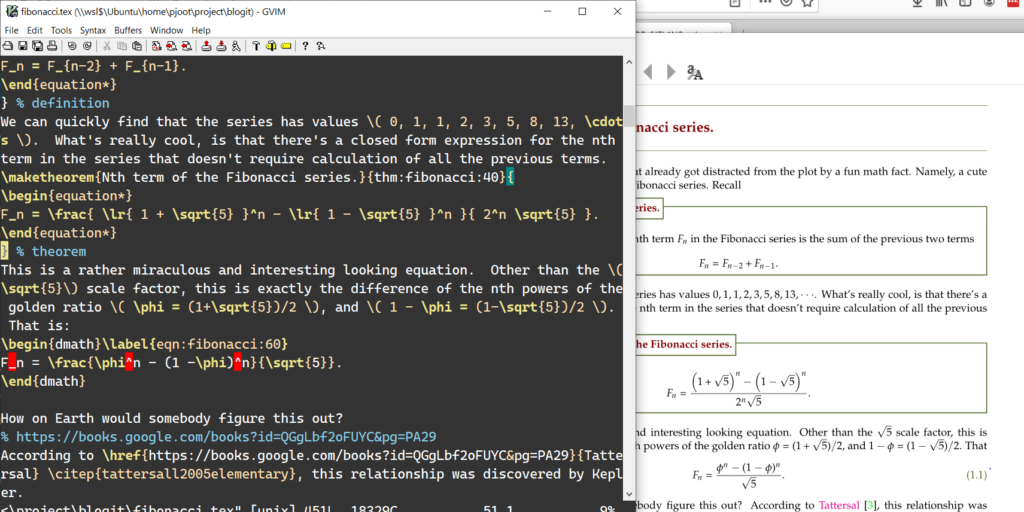I invoked the windows pdf viewer within a bash shell in the Ubuntu VM, using the following:


pjoot@DESKTOP-6J7L1NS:~/project/blogit$alias pdfview alias pdfview='/mnt/c/Users/peete/AppData/Local/SumatraPDF/SumatraPDF.exe' pjoot@DESKTOP-6J7L1NS:~/project/blogit$ pdfview fibonacci.pdf


The Ubuntu filesystem directory has the fibonacci.synctex.gz reverse lookup index that Summatra is able to read. Note that this file, after unzipping, has only Linux paths (/home/pjoot/…), but Summatra is able to use those without any trouble, and pops up the (Windows executable) editor on the files after I double click on the file. This sequence is pretty convoluted:

• Linux bash ->
• invoke Windows pdf viewer ->
• that program reading Linux files ->
• it invokes a windows editor (presumably using the Linux path), and that editor magically knows the path to the Linux file that it has to edit.

### C64 Nostalgia.

Incidentally, does anybody else still have their 6402 assembly programming references?  I’ve kept mine all these years, moving them around house to house, and taking a peek in them every few years, but I really ought to toss them!  I’m sure I couldn’t even give them away.

### Windows vs. MacOS

It’s been 4 years since I’ve actively used a Windows machine, and will have to relearn enough to get comfortable with it again (after suffering with the transition to MacOS and finally getting comfortable with it).  However, there are some new developments that I’m gung-ho to try, in particular, the new:

With WSL, I wonder if cygwin is even still a must have?  With windows terminal, I’m guessing that putty is a thing of the past (good riddance to cmd, that piece of crap.)

## Crashing Mathematica with HatchShading + Opacity

I attempted to modify a plot for an electric field solution that I had in my old Antenna-Theory notes:
\BE
=
j \omega
\frac{\mu_0 I_{\textrm{eo}} l}{4 \pi r} e^{-j k r}
\lr{ 1 + \cos\theta }
\lr{
-\cos\phi \thetacap
+ \sin\phi \phicap
},
\end{equation}
and discovered that you can crash Mathematica (12.1.0.0) by combining PlotStyle with Opacity and HatchShading (new in 12.1).  Here’s a stripped down version of the plot code that demonstrates the crash:

ClearAll[ rcap]
rcap = {Sin[#1] Cos[#2], Sin[#1] Sin[#2], Cos[#1]} & ;

{
ParametricPlot3D[
rcap[t, p]
, {t, 0, π}
, {p, 0, 2 π}
, PlotStyle -> { HatchShading[0.5, Black]}
]
, ParametricPlot3D[
rcap[t, p]
, {t, 0, π}
, {p, 0, 2 π}
, PlotStyle -> {Directive[Opacity[0.5]]}
], ParametricPlot3D[
rcap[t,p]
,{t,0,π}
,{p,0,2 π}
Black]}
]
}

The first two plots, using one, but not both of, Opacity or HatchShading work fine: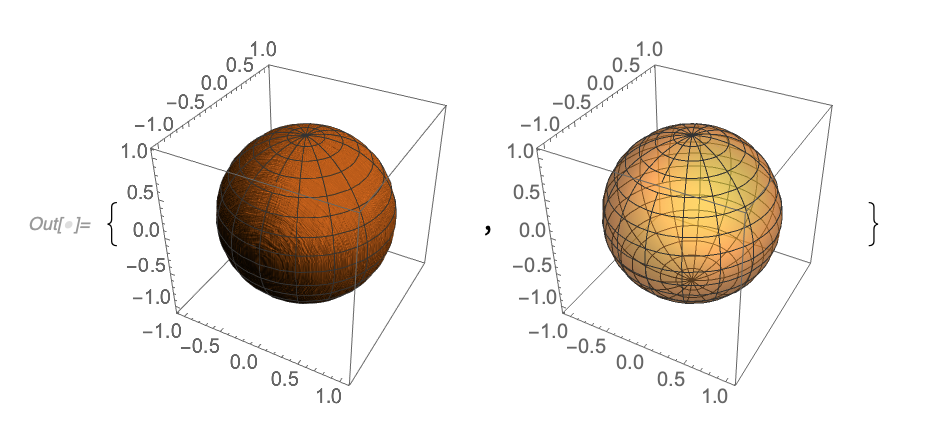In this reproducer, the little dimple at the base has been removed, which was the reason for the Opacity.

I’ve reported the bug to Wolfram, but wonder if they are going to come back to me saying, “Well, don’t do that!”

EDIT: Fixed in Mathematica 12.1.1

## Exploring 0^0, x^x, and z^z.

My Youtube home page knows that I’m geeky enough to watch math videos.  Today it suggested Eddie Woo’s video about $$0^0$$.

Mr Woo, who has great enthusiasm, and must be an awesome teacher to have in person.  He reminds his class about the exponent laws, which allow for an interpretation that $$0^0$$ would be equal to 1.  He points out that $$0^n = 0$$ for any positive integer, which admits a second contradictory value for $$0^0$$, if this was true for $$n=0$$ too.

When reviewing the exponent laws Woo points out that the exponent law for subtraction $$a^{n-n}$$ requires $$a$$ to be non-zero.  Given that restriction, we really ought to have no expectation that $$0^{n-n} = 1$$.

To attempt to determine a reasonable value for this question, resolving the two contradictory possibilities, neither of which we actually have any reason to assume are valid possibilities, he asks the class to perform a proof by calculator, computing a limit table for $$x \rightarrow 0+$$. I stopped at that point and tried it by myself, constructing such a table in Mathematica. Here is what I used

griddisp[labelc1_, labelc2_, f_, values_] := Grid[({
({{labelc1}, values}) // Flatten,
({ {labelc2}, f[#] & /@ values} ) // Flatten
}) // Transpose,
Frame -> All]
decimalFractions[n_] := ((10^(-#)) & /@ Range[n])
With[{m = 10}, griddisp[x, x^x, #^# &, N[decimalFractions[m], 10]]]
With[{m = 10}, griddisp[x, x^x, #^# &, -N[decimalFractions[m], 10]]]


Observe that I calculated the limits from both above and below. The results areand for the negative limitSure enough, from both below and above, we see numerically that $$\lim_{\epsilon\rightarrow 0} \epsilon^\epsilon = 1$$, as if the exponent law argument for $$0^0 = 1$$ was actually valid.  We see that this limit appears to be valid despite the fact that $$x^x$$ can be complex valued — that is ignoring the fact that a rigorous limit argument should be valid for any path neighbourhood of $$x = 0$$ and not just along two specific (real valued) paths.

Let’s get a better idea where the imaginary component of $$(-x)^{-x}$$ comes from.  To do so, consider $$f(z) = z^z$$ for complex values of $$z$$ where $$z = r e^{i \theta}$$. The logarithm of such a beast is

\begin{equation}\label{eqn:xtox:20}
\begin{aligned}
\ln z^z
&= z \ln \lr{ r e^{i\theta} } \\
&= z \ln r + i \theta z \\
&= e^{i\theta} \ln r^r + i \theta z \\
&= \lr{ \cos\theta + i \sin\theta } \ln r^r + i r \theta \lr{ \cos\theta + i \sin\theta } \\
&= \cos\theta \ln r^r – r \theta \sin\theta
+ i r \lr{ \sin\theta \ln r + \theta \cos\theta },
\end{aligned}
\end{equation}
so
\begin{equation}\label{eqn:xtox:40}
z^z =
e^{ r \lr{ \cos\theta \ln r – \theta \sin\theta}} \times
e^{i r \lr{ \sin\theta \ln r + \theta \cos\theta }}.
\end{equation}
In particular, picking the $$\theta = \pi$$ branch, we have, for any $$x > 0$$
\begin{equation}\label{eqn:xtox:60}
(-x)^{-x} = e^{-x \ln x – i x \pi } = \frac{e^{ – i x \pi }}{x^x}.
\end{equation}

Let’s get some visual appreciation for this interesting $$z^z$$ beastie, first plotting it for real values of $$z$$


Manipulate[
Plot[ {Re[x^x], Im[x^x]}, {x, -r, r}
, PlotRange -> {{-r, r}, {-r^r, r^r}}
, PlotLegends -> {Re[x^x], Im[x^x]}
], {{r, 2.25}, 0.0000001, 10}]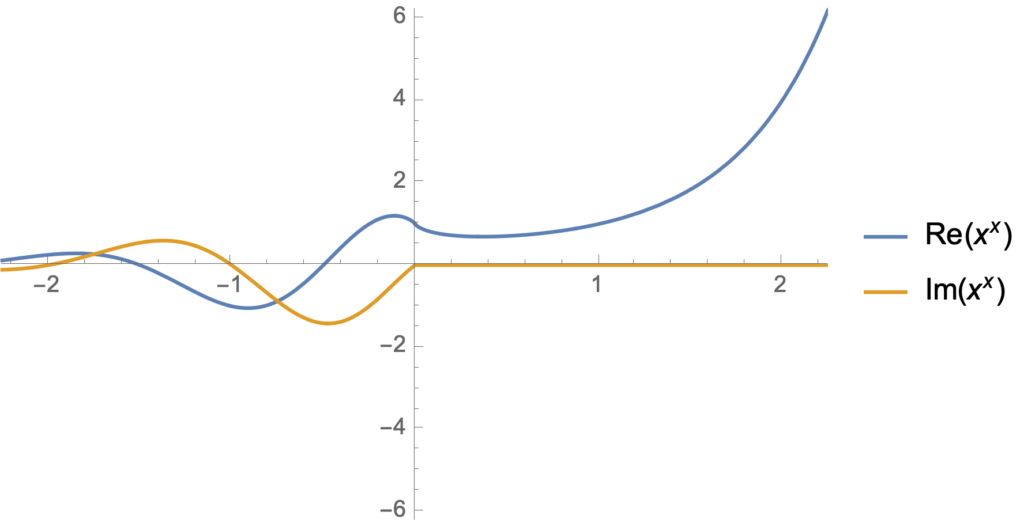From this display, we see that the imaginary part of $$x^x$$ is zero for integer values of $$x$$.  That’s easy enough to verify explicitly: $$(-1)^{-1} = -1, (-2)^{-2} = 1/4, (-3)^{-3} = -1/27, \cdots$$.

The newest version of Mathematica has a few nice new complex number visualization options.  Here’s two that I found illuminating, an absolute value plot that highlights the poles and zeros, also showing some of the phase action:

Manipulate[
ComplexPlot[ x^x, {x, s (-1 – I), s (1 + I)},
PlotLegends -> Automatic, ColorFunction -> "GlobalAbs"], {{s, 4},
0.00001, 10}]We see the branch cut nicely, the tendency to zero in the left half plane, as well as some of the phase periodicity in the regions that are in the intermediate regions between the zeros and the poles.  We can also plot just the phase, which shows its interesting periodic nature


Manipulate[
ComplexPlot[ x^x, {x, s (-1 – I), s (1 + I)},
PlotLegends -> Automatic, ColorFunction -> "CyclicArg"], {{s, 6},
0.00001, 10}]I’d like to take the time to play with some of the other ComplexPlot ColorFunction options, which appears to be a powerful and flexible visualization tool.

## Condensed matter physics notes

February 16, 2019 math and physics play No comments , , ,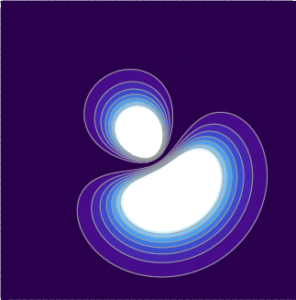Here’s an update of my old Condensed Matter Physics notes.

Condensed Matter

Along with a link to the notes, are instructions on building the PDF from the latex and the github clone commands required to make a copy of those sources.  Mathematica notebooks are also available for some of the calculations and plots.

## classical optics notes.

February 15, 2019 math and physics play No comments , , ,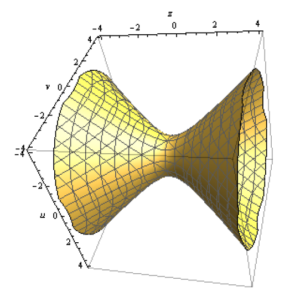Here’s an update of my old classical optics notes.  Along with a link to the notes, are instructions on building the PDF from the latex and the github clone commands required to make a copy of those sources.  Mathematica notebooks are also available for some of the calculations and plots.

Looks like most of the figures were hand drawn, but that was the only practical option, as this class was very visual.

## Mathematica notebooks updated, and a bivector addition visualization.

February 10, 2019 math and physics play No comments , , ,

This blog now has a copy of all my Mathematica notebooks (as of Feb 10, 2019), complete with a chronological index.  I hadn’t updated that index since 2014, and it was quite stale.

I’ve also added an additional level of per-directory indexing.  For example, you can now look at just the notebooks for my book, Geometric Algebra for Electrical Engineers.  That was possible before, but you would have had to clone the entire git repository to be able to do so easily.

This update includes a new notebook written today, which has a Manipulate visualization of 3D bivector addition that is kind of fun.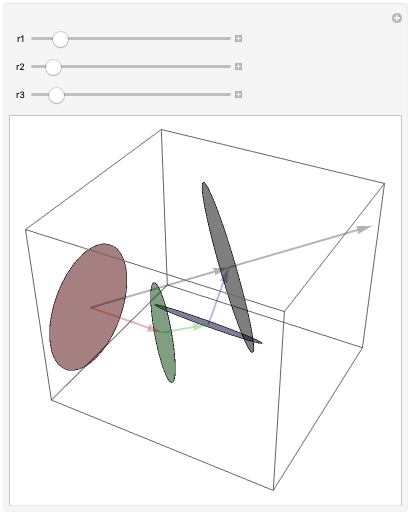Bivector addition, at least in 3D, can be done graphically almost like vector addition.  Instead of trying to add the planes (which can be done, as in the neat illustration in Geometric Algebra for Computer Science), you can do the task more simply by connecting the normals head to tail, where each of the normals are scaled by the area of the bivector (i.e. it’s absolute magnitude).  The resulting bivector has an area equal to the length of that sum of normals, and a “direction” perpendicular to that resulting normal.  This fun little Manipulate lets you interactively visualize this process, by changing the radius of a set of summed bivectors, each oriented in a different direction, and observing the effects of doing so.

Of course, you can interpret this visualization as nothing more than a representation of addition of cross products, if you were to interpret the vector representing a cross product as an oriented area with a normal equal to that cross product (where the normal’s magnitude equals the area, as in this bivector addition visualization.)  This works out nicely because of the duality relationship between the cross and wedge product, and the duality relationship between 3D bivectors and their normals.

## Electric field due to spherical shell

Here’s a problem (2.7) from , to calculate the field due to a spherical shell. The field is

\begin{equation}\label{eqn:griffithsEM2_7:20}
\BE = \frac{\sigma}{4 \pi \epsilon_0} \int \frac{(\Br – \Br’)}{\Abs{\Br – \Br’}^3} da’,
\end{equation}

where $$\Br’$$ is the position to the area element on the shell. For the test position, let $$\Br = z \Be_3$$. We need to parameterize the area integral. A complex-number like geometric algebra representation works nicely.

\begin{equation}\label{eqn:griffithsEM2_7:40}
\begin{aligned}
\Br’
&= R \lr{ \sin\theta \cos\phi, \sin\theta \sin\phi, \cos\theta } \\
&= R \lr{ \Be_1 \sin\theta \lr{ \cos\phi + \Be_1 \Be_2 \sin\phi } + \Be_3 \cos\theta } \\
&= R \lr{ \Be_1 \sin\theta e^{i\phi} + \Be_3 \cos\theta }.
\end{aligned}
\end{equation}

Here $$i = \Be_1 \Be_2$$ has been used to represent to horizontal rotation plane.

The difference in position between the test vector and area-element is

\begin{equation}\label{eqn:griffithsEM2_7:60}
\Br – \Br’
= \Be_3 \lr{ z – R \cos\theta } – R \Be_1 \sin\theta e^{i \phi},
\end{equation}

with an absolute squared length of

\begin{equation}\label{eqn:griffithsEM2_7:80}
\begin{aligned}
\Abs{\Br – \Br’ }^2
&= \lr{ z – R \cos\theta }^2 + R^2 \sin^2\theta \\
&= z^2 + R^2 – 2 z R \cos\theta.
\end{aligned}
\end{equation}

As a side note, this is a kind of fun way to prove the old “cosine-law” identity. With that done, the field integral can now be expressed explicitly

\begin{equation}\label{eqn:griffithsEM2_7:100}
\begin{aligned}
\BE
&= \frac{\sigma}{4 \pi \epsilon_0} \int_{\phi = 0}^{2\pi} \int_{\theta = 0}^\pi R^2 \sin\theta d\theta d\phi
\frac{\Be_3 \lr{ z – R \cos\theta } – R \Be_1 \sin\theta e^{i \phi}}
{
\lr{z^2 + R^2 – 2 z R \cos\theta}^{3/2}
} \\
&= \frac{2 \pi R^2 \sigma \Be_3}{4 \pi \epsilon_0} \int_{\theta = 0}^\pi \sin\theta d\theta
\frac{z – R \cos\theta}
{
\lr{z^2 + R^2 – 2 z R \cos\theta}^{3/2}
} \\
&= \frac{2 \pi R^2 \sigma \Be_3}{4 \pi \epsilon_0} \int_{\theta = 0}^\pi \sin\theta d\theta
\frac{ R( z/R – \cos\theta) }
{
(R^2)^{3/2} \lr{ (z/R)^2 + 1 – 2 (z/R) \cos\theta}^{3/2}
} \\
&= \frac{\sigma \Be_3}{2 \epsilon_0} \int_{u = -1}^{1} du
\frac{ z/R – u}
{
\lr{1 + (z/R)^2 – 2 (z/R) u}^{3/2}
}.
\end{aligned}
\end{equation}

Observe that all the azimuthal contributions get killed. We expect that due to the symmetry of the problem. We are left with an integral that submits to Mathematica, but doesn’t look fun to attempt manually. Specifically

\begin{equation}\label{eqn:griffithsEM2_7:120}
\int_{-1}^1 \frac{a-u}{\lr{1 + a^2 – 2 a u}^{3/2}} du
=
\left\{
\begin{array}{l l}
\frac{2}{a^2} & \quad \mbox{if $$a > 1$$ } \\
0 & \quad \mbox{if $$a < 1$$ } \end{array} \right., \end{equation} so \begin{equation}\label{eqn:griffithsEM2_7:140} \boxed{ \BE = \left\{ \begin{array}{l l} \frac{\sigma (R/z)^2 \Be_3}{\epsilon_0} & \quad \mbox{if $$z > R$$ } \\
0 & \quad \mbox{if $$z < R$$ } \end{array} \right. } \end{equation} In the problem, it is pointed out to be careful of the sign when evaluating $$\sqrt{ R^2 + z^2 - 2 R z }$$, however, I don't see where that is even useful?

# References

 David Jeffrey Griffiths and Reed College. Introduction to electrodynamics. Prentice hall Upper Saddle River, NJ, 3rd edition, 1999.

## Expectation of spherically symmetric 3D potential derivative

### Q:  pr 5.16

For a particle in a spherically symmetric potential $$V(r)$$ show that

\begin{equation}\label{eqn:symmetricPotentialDerivativeExpectation:20}
\Abs{\psi(0)}^2 = \frac{m}{2 \pi \Hbar^2} \expectation{ \frac{dV}{dr} },
\end{equation}

for all s-states, ground or excited.

Then show this is the case for the 3D SHO and hydrogen wave functions.

### A:

The text works a problem that looks similar to this by considering the commutator of an operator $$A$$, later set to $$A = p_r = -i \Hbar \PDi{r}{}$$ the radial momentum operator. First it is noted that

\begin{equation}\label{eqn:symmetricPotentialDerivativeExpectation:40}
0 = \bra{nlm} \antisymmetric{H}{A} \ket{nlm},
\end{equation}

since $$H$$ operating to either the right or the left is the energy eigenvalue $$E_n$$. Next it appears the author uses an angular momentum factoring of the squared momentum operator. Looking earlier in the text that factoring is found to be

\begin{equation}\label{eqn:symmetricPotentialDerivativeExpectation:60}
\frac{\Bp^2}{2m}
= \inv{2 m r^2} \BL^2 – \frac{\Hbar^2}{2m} \lr{ \PDSq{r}{} + \frac{2}{r} \PD{r}{} }.
\end{equation}

With
\begin{equation}\label{eqn:symmetricPotentialDerivativeExpectation:80}
R = – \frac{\Hbar^2}{2m} \lr{ \PDSq{r}{} + \frac{2}{r} \PD{r}{} }.
\end{equation}

we have

\begin{equation}\label{eqn:symmetricPotentialDerivativeExpectation:100}
\begin{aligned}
0
&= \bra{nlm} \antisymmetric{H}{p_r} \ket{nlm} \\
&= \bra{nlm} \antisymmetric{\frac{\Bp^2}{2m} + V(r)}{p_r} \ket{nlm} \\
&= \bra{nlm} \antisymmetric{\inv{2 m r^2} \BL^2 + R + V(r)}{p_r} \ket{nlm} \\
&= \bra{nlm} \antisymmetric{\frac{-\Hbar^2 l (l+1)}{2 m r^2} + R + V(r)}{p_r} \ket{nlm}.
\end{aligned}
\end{equation}

Let’s consider the commutator of each term separately. First

\begin{equation}\label{eqn:symmetricPotentialDerivativeExpectation:120}
\begin{aligned}
\antisymmetric{V}{p_r} \psi
&=
V p_r \psi

p_r V \psi \\
&=
V p_r \psi

(p_r V) \psi

V p_r \psi \\
&=

(p_r V) \psi \\
&=
i \Hbar \PD{r}{V} \psi.
\end{aligned}
\end{equation}

Setting $$V(r) = 1/r^2$$, we also have

\begin{equation}\label{eqn:symmetricPotentialDerivativeExpectation:160}
\antisymmetric{\inv{r^2}}{p_r} \psi
=
-\frac{2 i \Hbar}{r^3} \psi.
\end{equation}

Finally
\begin{equation}\label{eqn:symmetricPotentialDerivativeExpectation:180}
\begin{aligned}
\antisymmetric{\PDSq{r}{} + \frac{2}{r} \PD{r}{} }{ \PD{r}{}}
&=
\lr{ \partial_{rr} + \frac{2}{r} \partial_r } \partial_r

\partial_r \lr{ \partial_{rr} + \frac{2}{r} \partial_r } \\
&=
\partial_{rrr} + \frac{2}{r} \partial_{rr}

\lr{
\partial_{rrr} -\frac{2}{r^2} \partial_r + \frac{2}{r} \partial_{rr}
} \\
&=
-\frac{2}{r^2} \partial_r,
\end{aligned}
\end{equation}

so
\begin{equation}\label{eqn:symmetricPotentialDerivativeExpectation:200}
\antisymmetric{R}{p_r}
=-\frac{2}{r^2} \frac{-\Hbar^2}{2m} p_r
=\frac{\Hbar^2}{m r^2} p_r.
\end{equation}

Putting all the pieces back together, we’ve got
\begin{equation}\label{eqn:symmetricPotentialDerivativeExpectation:220}
\begin{aligned}
0
&= \bra{nlm} \antisymmetric{\frac{-\Hbar^2 l (l+1)}{2 m r^2} + R + V(r)}{p_r} \ket{nlm} \\
&=
i \Hbar
\bra{nlm} \lr{
\frac{\Hbar^2 l (l+1)}{m r^3} – \frac{i\Hbar}{m r^2} p_r +
\PD{r}{V}
}
\ket{nlm}.
\end{aligned}
\end{equation}

Since s-states are those for which $$l = 0$$, this means

\begin{equation}\label{eqn:symmetricPotentialDerivativeExpectation:240}
\begin{aligned}
\expectation{\PD{r}{V}}
&= \frac{i\Hbar}{m } \expectation{ \inv{r^2} p_r } \\
&= \frac{\Hbar^2}{m } \expectation{ \inv{r^2} \PD{r}{} } \\
&= \frac{\Hbar^2}{m } \int_0^\infty dr \int_0^\pi d\theta \int_0^{2 \pi} d\phi r^2 \sin\theta \psi^\conj(r,\theta, \phi) \inv{r^2} \PD{r}{\psi(r,\theta,\phi)}.
\end{aligned}
\end{equation}

Since s-states are spherically symmetric, this is
\begin{equation}\label{eqn:symmetricPotentialDerivativeExpectation:260}
\expectation{\PD{r}{V}}
= \frac{4 \pi \Hbar^2}{m } \int_0^\infty dr \psi^\conj \PD{r}{\psi}.
\end{equation}

That integral is

\begin{equation}\label{eqn:symmetricPotentialDerivativeExpectation:280}
\int_0^\infty dr \psi^\conj \PD{r}{\psi}
=
\evalrange{\Abs{\psi}^2}{0}{\infty} – \int_0^\infty dr \PD{r}{\psi^\conj} \psi.
\end{equation}

With the hydrogen atom, our radial wave functions are real valued. It’s reasonable to assume that we can do the same for other real-valued spherical potentials. If that is the case, we have

\begin{equation}\label{eqn:symmetricPotentialDerivativeExpectation:300}
2 \int_0^\infty dr \psi^\conj \PD{r}{\psi}
=
\Abs{\psi(0)}^2,
\end{equation}

and

\begin{equation}\label{eqn:symmetricPotentialDerivativeExpectation:320}
\boxed{
\expectation{\PD{r}{V}}
= \frac{2 \pi \Hbar^2}{m } \Abs{\psi(0)}^2,
}
\end{equation}

which completes this part of the problem.

### A: show this is the case for the 3D SHO and hydrogen wave functions

For a hydrogen like atom, in atomic units, we have

\begin{equation}\label{eqn:symmetricPotentialDerivativeExpectation:360}
\begin{aligned}
\expectation{
\PD{r}{V}
}
&=
\expectation{
\PD{r}{} \lr{ -\frac{Z e^2}{r} }
} \\
&=
Z e^2
\expectation
{
\inv{r^2}
} \\
&=
Z e^2 \frac{Z^2}{n^3 a_0^2 \lr{ l + 1/2 }} \\
&=
\frac{\Hbar^2}{m a_0} \frac{2 Z^3}{n^3 a_0^2} \\
&=
\frac{2 \Hbar^2 Z^3}{m n^3 a_0^3}.
\end{aligned}
\end{equation}

On the other hand for $$n = 1$$, we have

\begin{equation}\label{eqn:symmetricPotentialDerivativeExpectation:380}
\begin{aligned}
\frac{2 \pi \Hbar^2}{m} \Abs{R_{10}(0)}^2 \Abs{Y_{00}}^2
&=
\frac{2 \pi \Hbar^2}{m} \frac{Z^3}{a_0^3} 4 \inv{4 \pi} \\
&=
\frac{2 \Hbar^2 Z^3}{m a_0^3},
\end{aligned}
\end{equation}

and for $$n = 2$$, we have

\begin{equation}\label{eqn:symmetricPotentialDerivativeExpectation:400}
\begin{aligned}
\frac{2 \pi \Hbar^2}{m} \Abs{R_{20}(0)}^2 \Abs{Y_{00}}^2
&=
\frac{2 \pi \Hbar^2}{m} \frac{Z^3}{8 a_0^3} 4 \inv{4 \pi} \\
&=
\frac{\Hbar^2 Z^3}{4 m a_0^3}.
\end{aligned}
\end{equation}

These both match the potential derivative expectation when evaluated for the s-orbital ($$l = 0$$).

For the 3D SHO I verified the ground state case in the Mathematica notebook sakuraiProblem5.16bSHO.nb

There it was found that

\begin{equation}\label{eqn:symmetricPotentialDerivativeExpectation:420}
\expectation{\PD{r}{V}}
= \frac{2 \pi \Hbar^2}{m } \Abs{\psi(0)}^2
= 2 \sqrt{\frac{m \omega ^3 \Hbar}{ \pi }}
\end{equation}

# References

 Jun John Sakurai and Jim J Napolitano. Modern quantum mechanics. Pearson Higher Ed, 2014.

## L_y perturbation

### Q: $$L_y$$ perturbation.  pr. 5.17

Find the first non-zero energy shift for the perturbed Hamiltonian

\begin{equation}\label{eqn:LyPerturbation:20}
H = A \BL^2 + B L_z + C L_y = H_0 + V.
\end{equation}

### A:

The energy eigenvalues for state $$\ket{l, m}$$ prior to perturbation are

\begin{equation}\label{eqn:LyPerturbation:40}
A \Hbar^2 l(l+1) + B \Hbar m.
\end{equation}

The first order energy shift is zero

\begin{equation}\label{eqn:LyPerturbation:60}
\begin{aligned}
\Delta^1
&=
\bra{l, m} C L_y \ket{l, m} \\
&=
\frac{C}{2 i}
\bra{l, m} \lr{ L_{+} – L_{-} } \ket{l, m} \\
&=
0,
\end{aligned}
\end{equation}

so we need the second order shift. Assuming no degeneracy to start, the perturbed state is

\begin{equation}\label{eqn:LyPerturbation:80}
\ket{l, m}’ = \sum’ \frac{\ket{l’, m’} \bra{l’, m’}}{E_{l,m} – E_{l’, m’}} V \ket{l, m},
\end{equation}

and the next order energy shift is
\begin{equation}\label{eqn:LyPerturbation:100}
\begin{aligned}
\Delta^2
&=
\bra{l m} V
\sum’ \frac{\ket{l’, m’} \bra{l’, m’}}{E_{l,m} – E_{l’, m’}} V \ket{l, m} \\
&=
\sum’ \frac{\bra{l, m} V \ket{l’, m’} \bra{l’, m’}}{E_{l,m} – E_{l’, m’}} V \ket{l, m} \\
&=
\sum’ \frac{ \Abs{ \bra{l’, m’} V \ket{l, m} }^2 }{E_{l,m} – E_{l’, m’}} \\
&=
\sum_{m’ \ne m} \frac{ \Abs{ \bra{l, m’} V \ket{l, m} }^2 }{E_{l,m} – E_{l, m’}} \\
&=
\sum_{m’ \ne m} \frac{ \Abs{ \bra{l, m’} V \ket{l, m} }^2 }{
\lr{ A \Hbar^2 l(l+1) + B \Hbar m }
-\lr{ A \Hbar^2 l(l+1) + B \Hbar m’ }
} \\
&=
\inv{B \Hbar} \sum_{m’ \ne m} \frac{ \Abs{ \bra{l, m’} V \ket{l, m} }^2 }{
m – m’
}.
\end{aligned}
\end{equation}

The sum over $$l’$$ was eliminated because $$V$$ only changes the $$m$$ of any state $$\ket{l,m}$$, so the matrix element $$\bra{l’,m’} V \ket{l, m}$$ must includes a $$\delta_{l’, l}$$ factor.
Since we are now summing over $$m’ \ne m$$, some of the matrix elements in the numerator should now be non-zero, unlike the case when the zero first order energy shift was calculated above.

\begin{equation}\label{eqn:LyPerturbation:120}
\begin{aligned}
\bra{l, m’} C L_y \ket{l, m}
&=
\frac{C}{2 i}
\bra{l, m’} \lr{ L_{+} – L_{-} } \ket{l, m} \\
&=
\frac{C}{2 i}
\bra{l, m’}
\lr{
L_{+}
\ket{l, m}
– L_{-}
\ket{l, m}
} \\
&=
\frac{C \Hbar}{2 i}
\bra{l, m’}
\lr{
\sqrt{(l – m)(l + m + 1)} \ket{l, m + 1}

\sqrt{(l + m)(l – m + 1)} \ket{l, m – 1}
} \\
&=
\frac{C \Hbar}{2 i}
\lr{
\sqrt{(l – m)(l + m + 1)} \delta_{m’, m + 1}

\sqrt{(l + m)(l – m + 1)} \delta_{m’, m – 1}
}.
\end{aligned}
\end{equation}

After squaring and summing, the cross terms will be zero since they involve products of delta functions with different indices. That leaves

\begin{equation}\label{eqn:LyPerturbation:140}
\begin{aligned}
\Delta^2
&=
\frac{C^2 \Hbar}{4 B} \sum_{m’ \ne m} \frac{
(l – m)(l + m + 1) \delta_{m’, m + 1}

(l + m)(l – m + 1) \delta_{m’, m – 1}
}{
m – m’
} \\
&=
\frac{C^2 \Hbar}{4 B}
\lr{
\frac{ (l – m)(l + m + 1) }{ m – (m+1) }

\frac{ (l + m)(l – m + 1) }{ m – (m-1)}
} \\
&=
\frac{C^2 \Hbar}{4 B}
\lr{

(l^2 – m^2 + l – m)

(l^2 – m^2 + l + m)
} \\
&=
-\frac{C^2 \Hbar}{2 B} (l^2 – m^2 + l ),
\end{aligned}
\end{equation}

so to first order the energy shift is

\begin{equation}\label{eqn:LyPerturbation:160}
\boxed{
A \Hbar^2 l(l+1) + B \Hbar m \rightarrow
\Hbar l(l+1)
\lr{
A \Hbar
-\frac{C^2}{2 B}
}
+ B \Hbar m
+\frac{C^2 m^2 \Hbar}{2 B} .
}
\end{equation}

### Exact perturbation equation

If we wanted to solve the Hamiltonian exactly, we’ve have to diagonalize the $$2 m + 1$$ dimensional Hamiltonian

\begin{equation}\label{eqn:LyPerturbation:180}
\bra{l, m’} H \ket{l, m}
=
\lr{ A \Hbar^2 l(l+1) + B \Hbar m } \delta_{m’, m}
+
\frac{C \Hbar}{2 i}
\lr{
\sqrt{(l – m)(l + m + 1)} \delta_{m’, m + 1}

\sqrt{(l + m)(l – m + 1)} \delta_{m’, m – 1}
}.
\end{equation}

This Hamiltonian matrix has a very regular structure

\begin{equation}\label{eqn:LyPerturbation:200}
\begin{aligned}
H &=
(A l(l+1) \Hbar^2 – B \Hbar (l+1)) I \\
&+ B \Hbar
\begin{bmatrix}
1 & & & & \\
& 2 & & & \\
& & 3 & & \\
& & & \ddots & \\
& & & & 2 l + 1
\end{bmatrix} \\
&+
\frac{C \Hbar}{i}
\begin{bmatrix}
0 & -\sqrt{(2l-1)(1)} & & & \\
\sqrt{(2l-1)(1)} & 0 & -\sqrt{(2l-2)(2)}& & \\
& \sqrt{(2l-2)(2)} & & & \\
& & \ddots & & \\
& & & 0 & – \sqrt{(1)(2l-1)} \\
& & & \sqrt{(1)(2l-1)} & 0
\end{bmatrix}
\end{aligned}
\end{equation}

Solving for the eigenvalues of this Hamiltonian for increasing $$l$$ in Mathematica (sakuraiProblem5.17a.nb), it appears that the eigenvalues are

\begin{equation}\label{eqn:LyPerturbation:220}
\lambda_m = A \Hbar^2 (l)(l+1) + \Hbar m B \sqrt{ 1 + \frac{4 C^2}{B^2} },
\end{equation}

so to first order in $$C^2$$, these are

\begin{equation}\label{eqn:LyPerturbation:221}
\lambda_m = A \Hbar^2 (l)(l+1) + \Hbar m B \lr{ 1 + \frac{2 C^2}{B^2} }.
\end{equation}

We have a $$C^2 \Hbar/B$$ term in both the perturbative energy shift, and the first order expansion of the exact solution. Comparing this for the $$l = 5$$ case, the coefficients of $$C^2 \Hbar/B$$ in the perturbative solution are all negative $$-17.5, -17., -16.5, -16., -15.5, -15., -14.5, -14., -13.5, -13., -12.5$$, whereas the coefficient of $$C^2 \Hbar/B$$ in the first order expansion of the exact solution are $$2 m$$, ranging from $$[-10, 10]$$.

# References

 Jun John Sakurai and Jim J Napolitano. Modern quantum mechanics. Pearson Higher Ed, 2014.